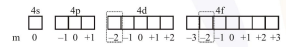# The number of subshells associated with

Question:

The number of subshells associated with $\mathrm{n}=4$ and $m=-2$ quantum numbers is:

1. 4

2. 8

3. 16

4. 2

Correct Option: , 4

Solution:

For $n=4$

$\ell=0,1,2,3$$\therefore 4 \mathrm{~d} \& 4 \mathrm{f}$ subshell associated with $\mathrm{n}=4, \mathrm{~m}=-2$